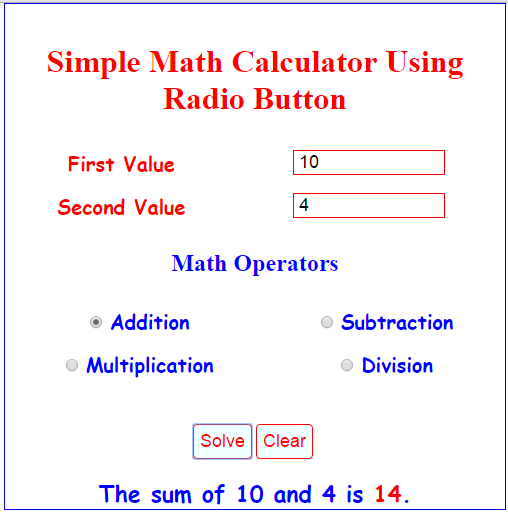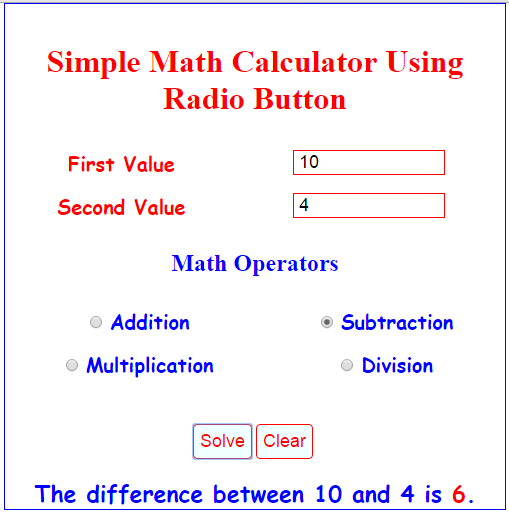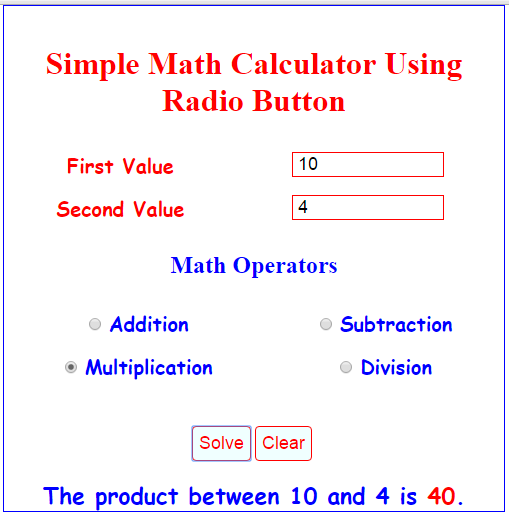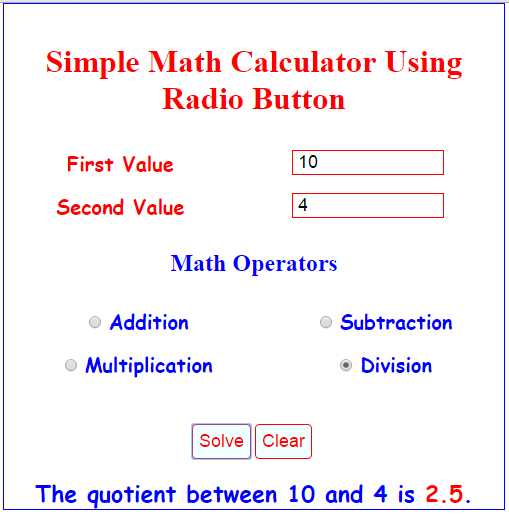# Simple Math Calculator Using Radio Button

Related Code: Math Calculator Using CheckBox

This is a simple program using JavaScript. And it’s called Simple Math Calculator Using Radio Button. This is a very common program, but this is suited for the beginner in JavaScript Language.

This program contains 2 textboxes where the user types for the numeric value, then 4 radio button for addition, subtraction, multiplication, and division to select the arithmetic operators. And we have 1 button to compute and 1 button for clearing the value in the textbox to solve another numeric value.

## Math Operators

• Subtraction
• Multiplication
• Division

JavaScript Source Code

This script will execute after the user controls the form field.

`<script>function solve() { var operators_If = document.getElementsByName("math_Operators");var first_TextBox_Value = document.getElementById("number1_TextBox").value;var no_two = document.getElementById("number2_TextBox").value;  if (first_TextBox_Value=="") {var math_Results = "<span class='warning'>Enter the first value.</span>";document.getElementById('math_Results').innerHTML = math_Results;return false;} else if (no_two=="") {var math_Results = "<span class='warning'>Enter the second value.</span>";document.getElementById('math_Results').innerHTML = math_Results;return false;} if (operators_If.checked == true) {sum = parseInt(first_TextBox_Value) + parseInt(no_two);							results = "<span class='message'> The sum of " + first_TextBox_Value + " and " + no_two + " is <b style='color:red;'>" + sum +"</b>.</span>";document.getElementById('math_Results').innerHTML = results;return false;} else if (operators_If.checked == true) { diff = parseInt(first_TextBox_Value) - parseInt(no_two);					results = "<span class='message'> The difference between " + first_TextBox_Value + " and " + no_two + " is <b style='color:red;'>" + diff +"</b>.</span>";document.getElementById('math_Results').innerHTML = results;return false;} else if (operators_If.checked == true) { product = parseInt(first_TextBox_Value) *parseInt(no_two);					results = "<span class='message'>The product between " + first_TextBox_Value + " and " + no_two + " is <b style='color:red;'>" + product +"</b>.</span>";document.getElementById('math_Results').innerHTML = results;return false;} else if (operators_If.checked == true) { quotient = parseInt(first_TextBox_Value) / parseInt(no_two);results = "<span class='message'>The quotient between " + first_TextBox_Value + " and " + no_two + " is <b style='color:red;'>" + quotient +"</b>. </span>";document.getElementById('math_Results').innerHTML = results;return false;} else {var math_Results = "<span class='warning'>Select your math operators.</span>";document.getElementById('math_Results').innerHTML = math_Results;return false;}return true;}function reset_Operators() {document.getElementById('math_Results').innerHTML = '';}function clear(){document.getElementById('math_Results').innerHTML = "";first_TextBox_Value="";no_two="";first_TextBox_Value.focus();}</script>`

HTML Form Field

This form field contains textboxes, radio button, and buttons to operate this program.

`<form action="" method="POST"><table cellpadding="5" cellspacing="5" style="text-align:center;" width="100%" border="0">	<tr>		<td><label>First Value</label></td>		<td><input type="number" name="first_TextBox_Value" id="number1_TextBox" autofocus="autofocus"></td>	</tr>	<tr>		<td><label>Second Value</label></td>		<td><input type="number" name="second_TextBox_Value" id="number2_TextBox"></td>	</tr></table><h2>Math Operators</h2><table cellpadding="5" cellspacing="5" style="text-align:center;" width="100%" border="0">	<tr>		<td><input type="radio" name="math_Operators" onclick="reset_Operators();" />   <label style="color:blue;">Addition</label></td>		<td><input type="radio" name="math_Operators" onclick="reset_Operators();" />   <label style="color:blue;">Subtraction</label></td>	</tr>	<tr>		<td><input type="radio" name="math_Operators" onclick="reset_Operators();" />   <label style="color:blue;">Multiplication</label></td>		<td><input type="radio" name="math_Operators" onclick="reset_Operators();" />   <label style="color:blue;">Division</label></td>	</tr></table><br /><br />           <input type="submit" value="Solve" onclick="return solve();" /><input type="submit" value="Clear" onclick="return clear();" /><br /><br /><div id="math_Results"></div></form>`

And, the style.

`<style type="text/css">body{	width:500px;	margin:auto;	border:blue 1px solid;	text-align:center;}h1 {	color:red;}h2 {	color:blue;}label {    font-size: 20px;    font-weight: bolder;    font-family: cursive;    color: red;}input[type="number"] {    font-size: 18px;    border: red 1px solid;    text-indent: 5px;    width: 150px;}input[type="submit"] {    font-size: 18px;    color: red;    background: azure;    border: red 1px solid;    padding: 6px;    border-radius: 4px;	cursor:pointer;}#math_Results{	font-size: 23px;    font-weight: bold;    font-family: cursive;    color: blue;}</style>`

## Output:For Subtraction Operator.For Multiplication Operator.For Division Operator.Related Code: Math Calculator Using CheckBox

So, this is it, you have Simple Math Calculator Using Radio Button in JavaScript. Kindly click the "Download Code" button for full source code. Enjoy Coding. Hope you can learn from this.

Share us your thoughts and comments below. Thank you so much for dropping by and reading this tutorial post. For more updates, don’t hesitate and feel free to visit this website more often and please share this with your friends or email me at [email protected]. Practice Coding. Thank you very much.

### Tags

Note: Due to the size or complexity of this submission, the author has submitted it as a .zip file to shorten your download time. After downloading it, you will need a program like Winzip to decompress it.

Virus note: All files are scanned once-a-day by SourceCodester.com for viruses, but new viruses come out every day, so no prevention program can catch 100% of them.